# AP Calculus AB : Implicit differentiation

## Example Questions

1 2 4 Next →

### Example Question #84 : Applications Of Derivatives

Find the normal line of the curve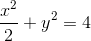at the point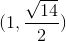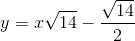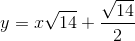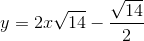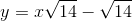Explanation:Use implicit differentiation to calculate the slope of the tangent line: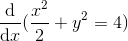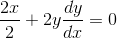Simplify: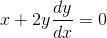Subtract x from both sides of the equation: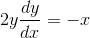Divide both sides of the equation by 2y: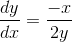Plug in the x and y values from the pointinto the equation, to calculate the slope of the tangent line: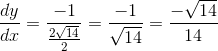Take the negative reciprocal of the slope of the tangent line to calculate the slope of the normal line: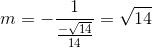Plug the slope of the normal line into point slope form: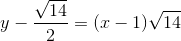Add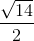to both sides to isolate y: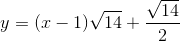Simplify the equation: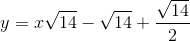Simplify further:Solution:1 2 4 Next →